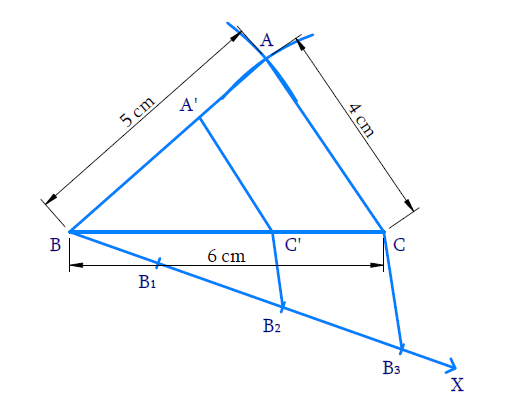# Ex.11.1 Q2 Constructions Solution - NCERT Maths Class 10

Go back to  'Ex.11.1'

## Question

Construct a triangle of sides $$4 \;\rm{cm}$$, $$5 \;\rm{cm}$$ and $$6\; \rm{cm}$$ and then a triangle similar to it whose sides are \begin{align}\frac{2}{3}\end{align} of the corresponding sides of the first triangle.

Video Solution
Constructions
Ex 11.1 | Question 2

## Text Solution

#### What is known?

Sides of the triangle and the ratio of corresponding sides of $$2$$ triangles.

Construction.

#### Reasoning:

• Draw the line segment of largest length $$6\, \rm{cm}$$. Measure $$5 \;\rm{cm}$$ and $$4 \;\rm{cm}$$ separately and cut arcs from $$2$$ ends of the line segment such that they cross each other at one point. Connect this point from both the ends.
• Then draw another line which makes an acute angle with the given line ($$6\; \rm{cm}$$).
• Divide the line into $$(m + n)$$ parts where $$m$$ and $$n$$ are the ratio given.
• Two triangles are said to be similar if their corresponding angles are equal. They are said to satisfy Angle-Angle-Angle (AAA) Axiom.
• Basic proportionality theorem states that, “If a straight line is drawn parallel to a side of a triangle, then it divides the other two sides proportionally".

#### Steps:Steps of constructions:

(i) Draw $$BC = 6 \text{cm.}$$ With $$B$$ and $$C$$ as centres and radii $$5 \;\rm{cm}$$ and $$4\,\rm{cm}$$ respectively draw arcs to intersect at $$A.$$  $${\rm{\Delta ABC}}$$ is obtained.

(ii) Draw ray $$BX$$ making an acute angle with $$BC.$$

(iii) Mark $$3$$ ( {$$3> 2$$ in the ratio \begin{align}\frac{2}{3}\end{align}points $${{{B}}_{{1}}}{{,}}\,\,{{{B}}_{{2}}}{{,}}\,\,{{{B}}_{{3}}}$$ on $$BX$$ such that $$\,{{B}}{{{B}}_{{1}}}{{ = }}{{{B}}_{{1}}}{{{B}}_{{2}}}{{ = }}{{{B}}_{{2}}}{{{B}}_{{3}}}$$ .

(iv) Join $$\,{{{B}}_{\rm{3}}}{{C}}$$ and draw the line through $${B_2}$$  (2nd point where $$2 < 3$$ in the ratio \begin{align}\frac{2}{3}\end{align}) parallel to $$B_3C$$ meeting $$BC$$ at $${{C'.}}$$

(v) Draw a line thorough $$\,{{C'}}$$ parallel to $$CA$$ to meet $$BA$$ at $$A’.$$ Now $$\,{{\Delta A'BC'}}$$ is the required triangle similar to $$\,{{\Delta ABC}}$$ where

\begin{align}\frac{{{{BC'}}}}{{{{BC}}}}&{{ = }}\frac{{{{BA'}}}}{{{\rm{BA}}}}{\rm{ = }}\frac{{{{C'A'}}}}{{{\rm{CA}}}}\\&{{ = }}\frac{{{2}}}{{{3}}}\end{align}

Proof:

In $$\Delta B B_{3} C, B_{2} C$$ is parallel to $$\,{{{B}}_{{3}}}{{C}} .$$

Hence by Basic proportionality theorem,

\begin{align}\frac{{{{{B}}_{{2}}}{{{B}}_{{2}}}}}{{{{B}}{{{B}}_{{2}}}}}&{{ = }}\frac{{{{C'C}}}}{{{{BC'}}}}\\&{{ = }}\frac{{{1}}}{{{2}}}\end{align}

Adding $$1$$,

\begin{align}\frac{{{{C'C}}}}{{{{BC'}}}}{{ + 1}}& = \frac{{{1}}}{{{2}}}{{ + 1}}\\\frac{{{{C'C + BC'}}}}{{{{BC'}}}}{{ }}&=\frac{{{3}}}{{{2}}}\\\,\,\,\,\,\,\,\,\,\,\,\,\,\,\frac{{{{BC}}}}{{{{BC'}}}}{{}}&= \frac{{{3}}}{{{2}}}\\\,\,\,\,\,\text{(or)}\,\,\,\,\,\,\frac{{{{BC'}}}}{{{{BC}}}}{{ }}&=\frac{{{2}}}{{{3}}} & & {{ \ldots (1)}}\end{align}

Consider $${\rm{\Delta BA'C'}}$$ and $${\rm{\Delta BAC}}$$

$$\angle \text{A }\!\!'\!\!\text{ BC }\!\!'\!\!\text{ }=\angle \text{ABC}$$ (Common)

$$\angle \text{BA }\!\!'\!\!\text{ C }\!\!'\!\!\text{ }=\angle \text{BAC}$$ (Corresponding angles $$∵$$  $$\,\,\text{C }\!\!'\!\!\text{ }A'||\,\text{CA}$$ )

$$\angle \text{BA }\!\!'\!\!\text{ C }\!\!'\!\!\text{ }=\angle \text{BCA}$$ (Corresponding angles $$∵$$ $$\,\,\text{C }\!\!'\!\!\text{ }A'||CA$$ )

Hence by AAA axiom,

${{\Delta BA'C' \sim \Delta BAC}}\,\,$

Corresponding sides are proportional

\begin{align}\frac{{{{BA'}}}}{{{{BA}}}}{{ = }}\frac{{{{C'A'}}}}{{{{CA}}}}{{ = }}\frac{{{{BC'}}}}{{{{BC}}}}{{ = }}\frac{{{2}}}{{{3}}}\,\,\left( {{\text{from}}\,{{(1)}}} \right)\end{align}

Learn from the best math teachers and top your exams

• Live one on one classroom and doubt clearing
• Practice worksheets in and after class for conceptual clarity
• Personalized curriculum to keep up with school